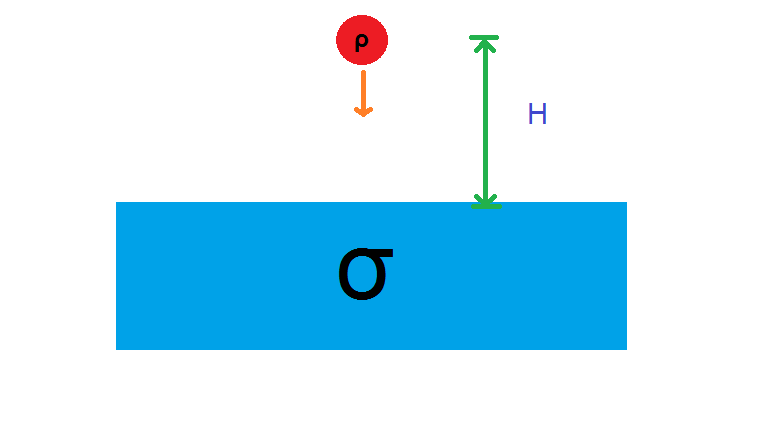# Ball FallA ball of density $\rho$ is dropped from rest at a height $H$ into a lake of water with density $\sigma$ such that $\sigma > \rho$. Neglecting damping forces, calculate the maximum depth to which the body sinks before turning around.

Assumptions

• $H\gg r_{\rm{ball}}$, i.e. the ball has some infinitesimal volume $dV$.
×

Problem Loading...

Note Loading...

Set Loading...